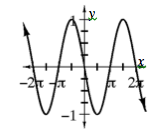### Home > A2C > Chapter 13 > Lesson 13.2.3 > Problem13-132

13-132.

Consider the function $f\left(x\right)=\cos\left(x+\frac{\pi}{2}\right)$.

1. Make a complete graph of $f(x)$.2. Write a simpler trig function that is represented by the same graph.

Which trig function passes through the origin?

3. Now use the angle sum identity to expand and simplify $\cos\left(x+\frac{\pi}{2}\right)$.

Use Angle Sum Identities.

4. Does your work in part (c) agree with your graphical results from parts (a) and (b)? Explain.